﻿ 基于CFD的玻璃温室环境数值模拟及优化分析
«上一篇文章快速检索 高级检索

 应用科技2019, Vol. 46Issue (5): 28-33  DOI: 10.11991/yykj.2019010050

### 引用本文JIA Heming, HAN Juncheng, ZHANG Sen, et al. Numerical analysis simulation and optimization of the environment in glass greenhouse based on CFD[J]. Applied Science and Technology, 2019, 46(5), 28-33. DOI: 10.11991/yykj.201901005.### 文章历史

Numerical analysis simulation and optimization of the environment in glass greenhouse based on CFD
JIA Heming, HAN Juncheng , ZHANG Sen , SUN Kangjian , LI Yao
College of Mechanical and Electrical Engineering, Northeast Forestry University, Harbin 150040, China
Abstract: In order to further study and optimize the distribution of air flow and temperature field in glass greenhouse, a numerical analysis of the three-dimensional modeling of air flow field in glass greenhouse in the Northeast China based on computational fluid dynamics (CFD) is carried out in this paper. Then the distribution patterns of internal velocity field and temperature field are derived, and three structural optimization strategies are put forward according to the distribution of air flow in glass greenhouse. The simulation results show that the average relative errors of gas velocity and temperature at the monitoring points are 5.54% and 4%, respectively. The numerical simulation results are in good agreement with the experimental results. The results of these three optimization schemes are better than the initial ones. The best scheme is the third one, in which the warm fan is placed at 0.7 m away from the ground. The optimum wind speed is increased by 50% in the plant area. The air flow uniformity is the best and the average temperature is 23.49 ℃. It can effectively improve the greenhouse environment and make it more suitable for plant cultivation.
Keywords: CFD    glass greenhouse    air movement    temperature field    velocity field    porous medium    numerical simulation    uniformity    structure optimization

1 材料和方法 1.1 温室模型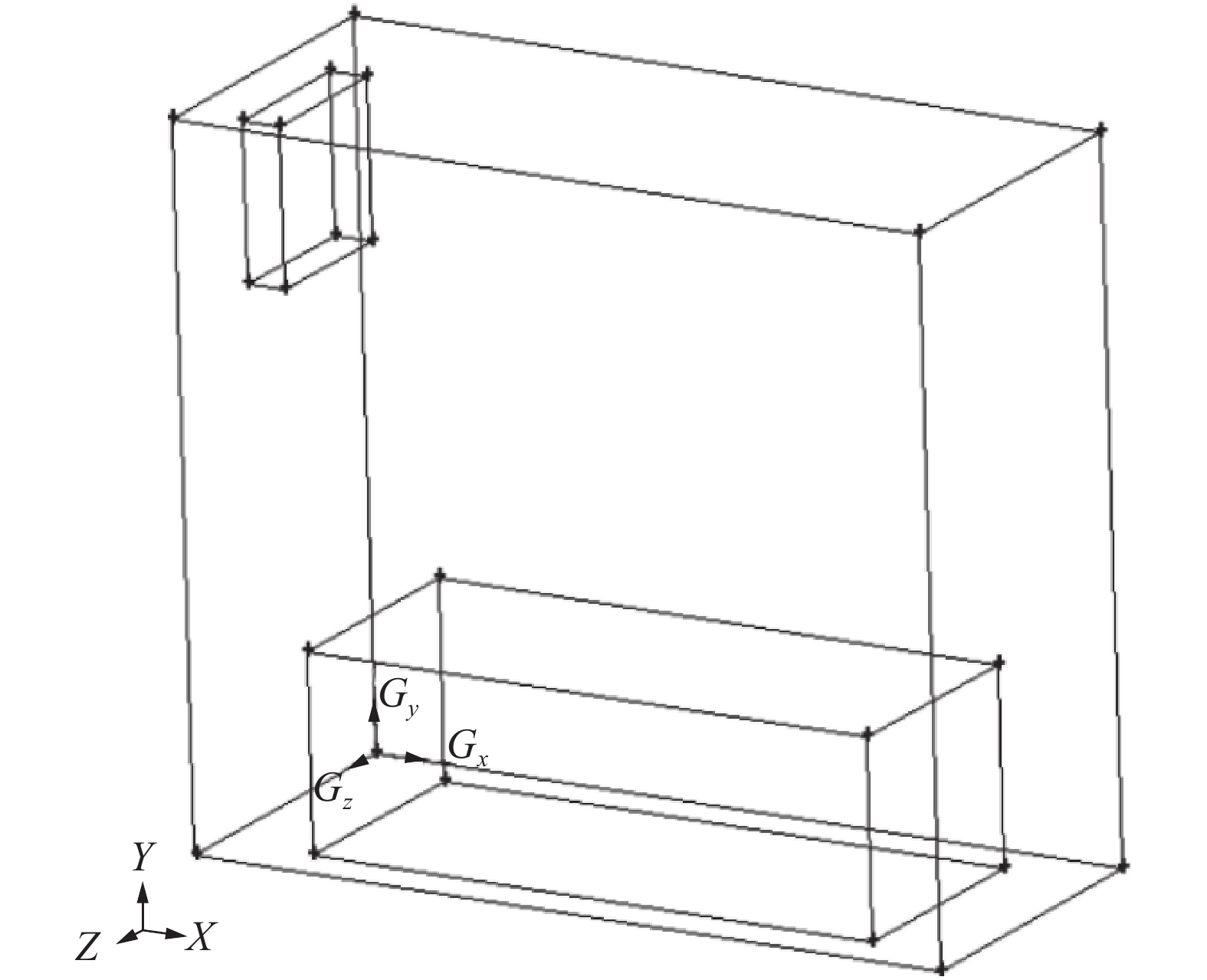Download: 图 1 玻璃温室模型
1.2 监测点布置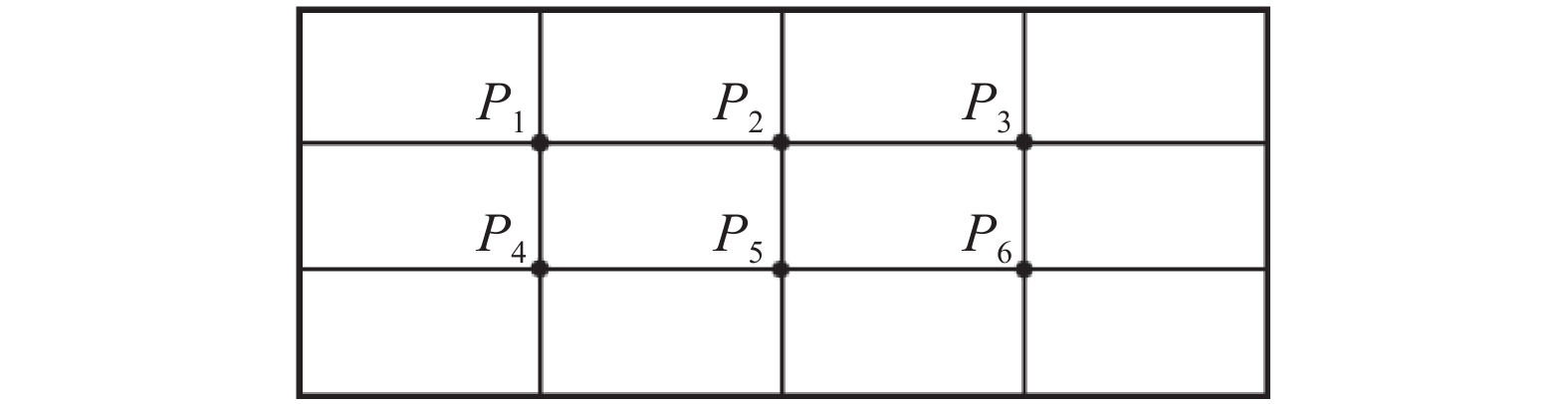Download: 图 2 Z=0.415 m截面监测点布置（Y=0.6 m和Y=1.2 m）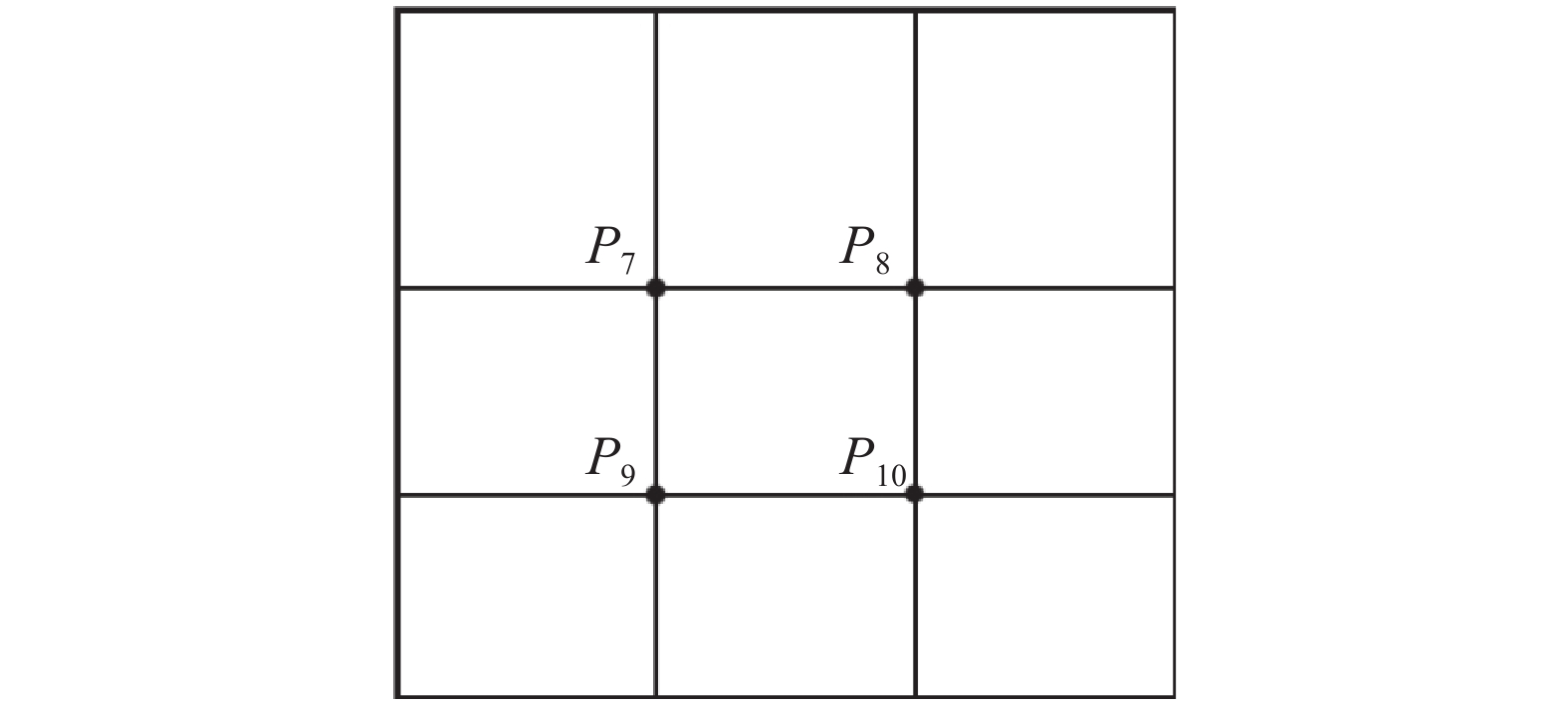Download: 图 3 Z=0.415 m截面监测点布置（X=1 m）
1.3 数学模型 1.3.1 CFD模型

 $\frac{{\partial u}}{{\partial x}} + \frac{{\partial v}}{{\partial y}} + \frac{{\partial w}}{{\partial z}} = 0$

 $\begin{gathered} \frac{{\partial (\rho uu)}}{{\partial x}} + \frac{{\partial (\rho uv)}}{{\partial y}} + \frac{{\partial (\rho uw)}}{{\partial z}} = - \frac{{\partial P}}{{\partial x}} + \\ {\mu _{{\rm{eff}}}}\left( {\frac{{{\partial ^2}u}}{{\partial {x^2}}} + \frac{{{\partial ^2}u}}{{\partial {y^2}}} + \frac{{{\partial ^2}u}}{{\partial {z^2}}}} \right) \\ \end{gathered}$
 $\begin{gathered} \frac{{\partial (\rho vu)}}{{\partial x}} + \frac{{\partial (\rho vv)}}{{\partial y}} + \frac{{\partial (\rho vw)}}{{\partial z}} = - \frac{{\partial P}}{{\partial y}} + \\ {\mu _{{\rm{eff}}}}\left( {\frac{{{\partial ^2}v}}{{\partial {x^2}}} + \frac{{{\partial ^2}v}}{{\partial {y^2}}} + \frac{{{\partial ^2}v}}{{\partial {z^2}}}} \right){\rm{ - }}{\rho _{{\rm{ref}}}}g[1 - \beta (T - T{\rm{ref}})] \\ \end{gathered}$
 $\begin{gathered} \frac{{\partial (\rho wu)}}{{\partial x}} + \frac{{\partial (\rho wv)}}{{\partial y}} + \frac{{\partial (\rho ww)}}{{\partial z}} = - \frac{{\partial P}}{{\partial z}} + \\ {\mu _{{\rm{eff}}}}\left( {\frac{{{\partial ^2}w}}{{\partial {x^2}}} + \frac{{{\partial ^2}w}}{{\partial {y^2}}} + \frac{{{\partial ^2}w}}{{\partial {z^2}}}} \right) \\ \end{gathered}$

 $\begin{gathered} \frac{{\partial (\rho uT)}}{{\partial x}} + \frac{{\partial (\rho vT)}}{{\partial y}} + \frac{{\partial (\rho wT)}}{{\partial z}} = - \frac{q}{{{C_P}}} + \\ \frac{{{\lambda _{{\rm{eff}}}}}}{{{C_p}}} + \left( {\frac{{{\partial ^2}T}}{{\partial {x^2}}} + \frac{{{\partial ^2}T}}{{\partial {y^2}}} + \frac{{{\partial ^2}T}}{{\partial {z^2}}}} \right) \\ \end{gathered}$

 $\rho {\rm{ = }}{\rho _{\rm{0}}}\left[ {{\rm{1 - }}\beta \left( {T - {T_0}} \right)} \right]$

 ${S_\varphi } = - \left( {\frac{\mu }{{{K_p}}} + \frac{{{C_F}}}{{\sqrt {{K_p}} }}\rho {u^2}} \right)$

 ${S_\varphi } = - {I_{{\rm{LAV}}}}{C_D}\rho {u^2}$

1.3.2 计算域与网格划分

1.3.3 边界条件

1.3.4 求解运算

2 FLUENT仿真结果与验证 2.1 FLUENT仿真结果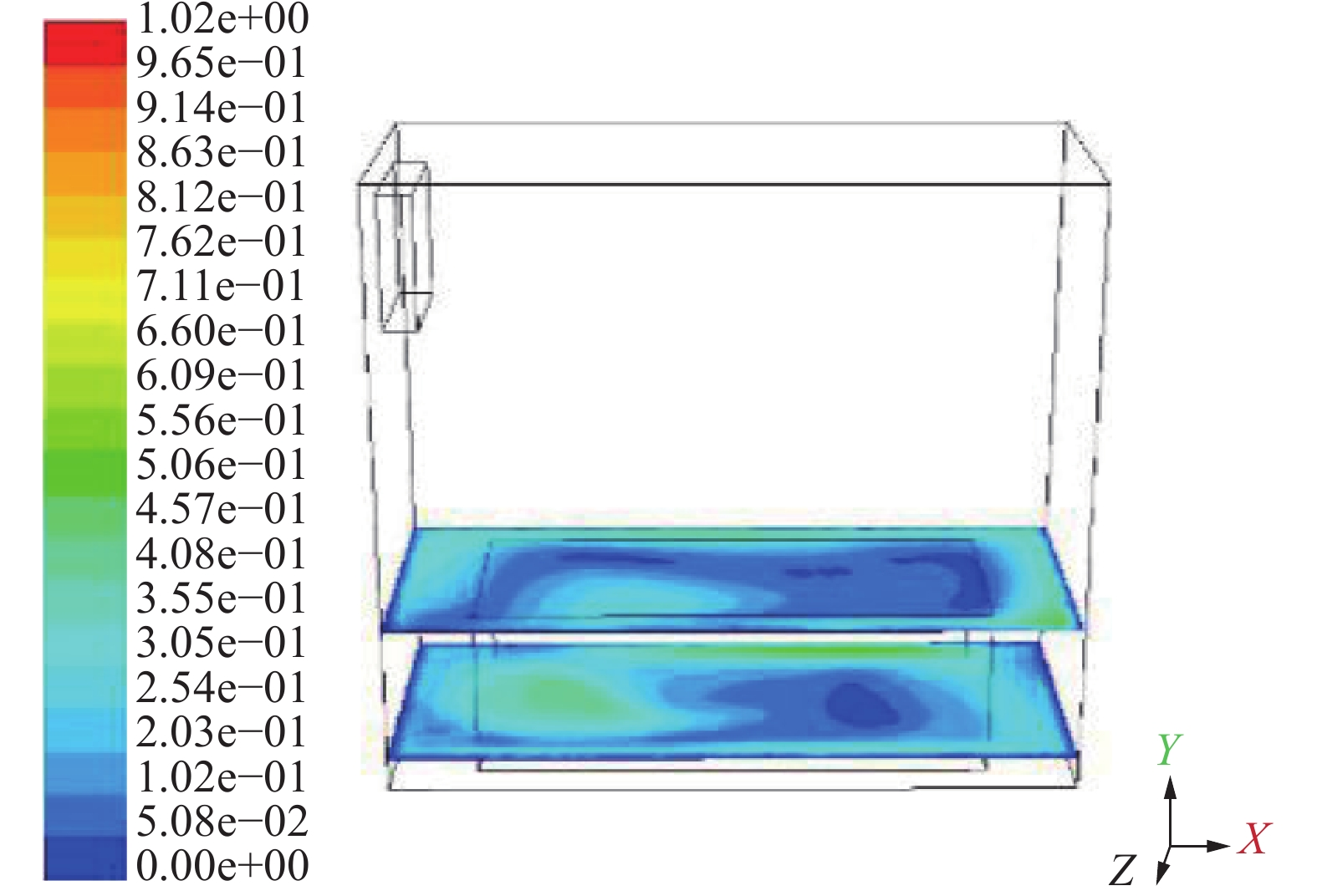Download: 图 4 Y=0.5 m和Y=0.1 m处速度云图Download: 图 5 Y=0.5 m和Y=0.1 m处温度云图

2.2 对比验证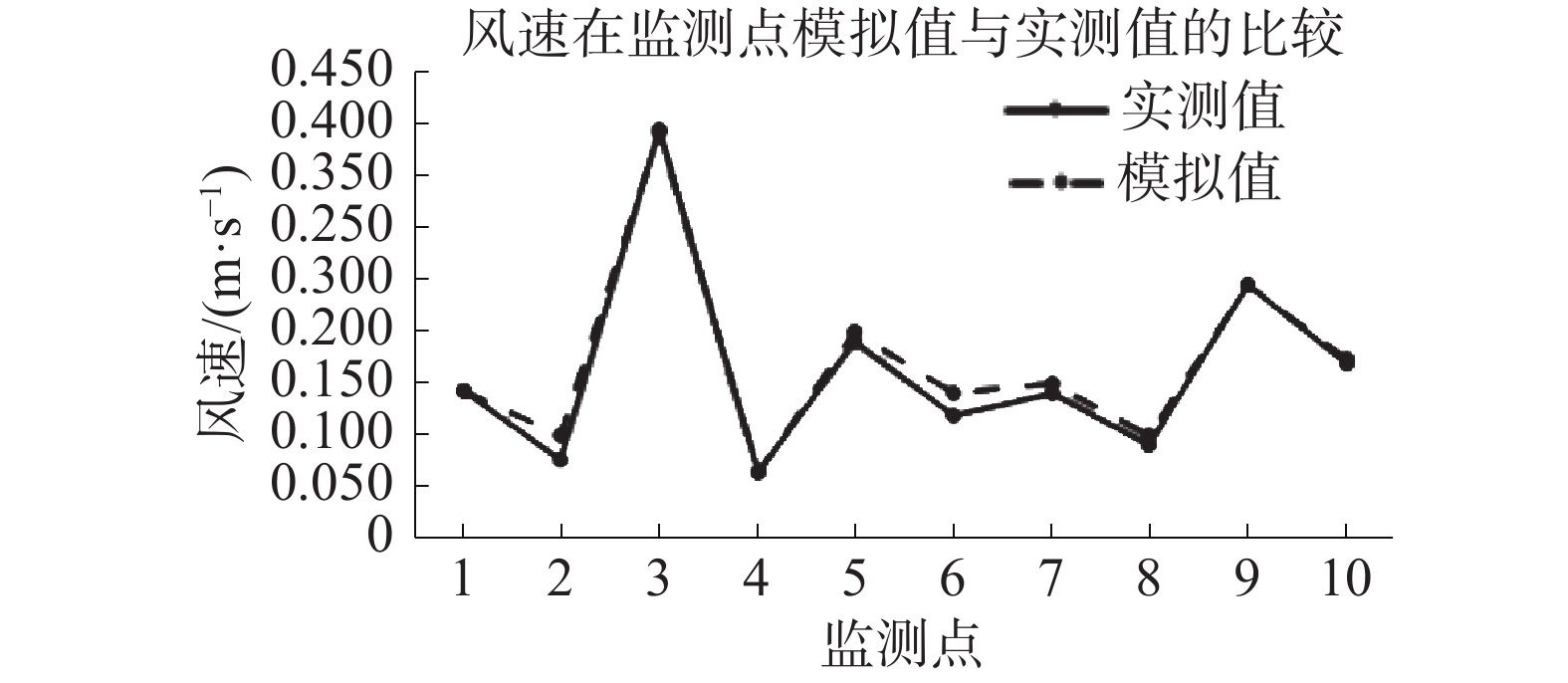Download: 图 6 风速在监测点模拟值与实测值的比较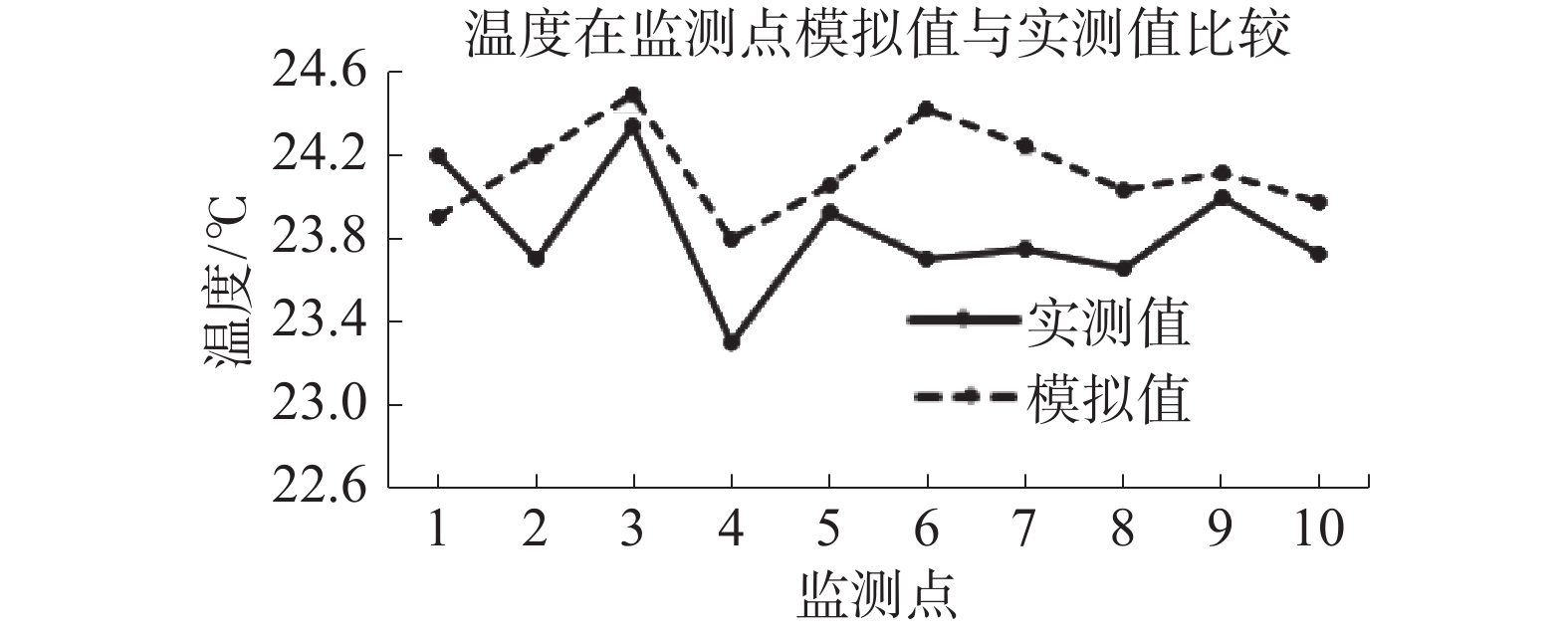Download: 图 7 温度在监测点模拟值与实测值比较

3 温室内环境的优化设计 3.1 优化方案设计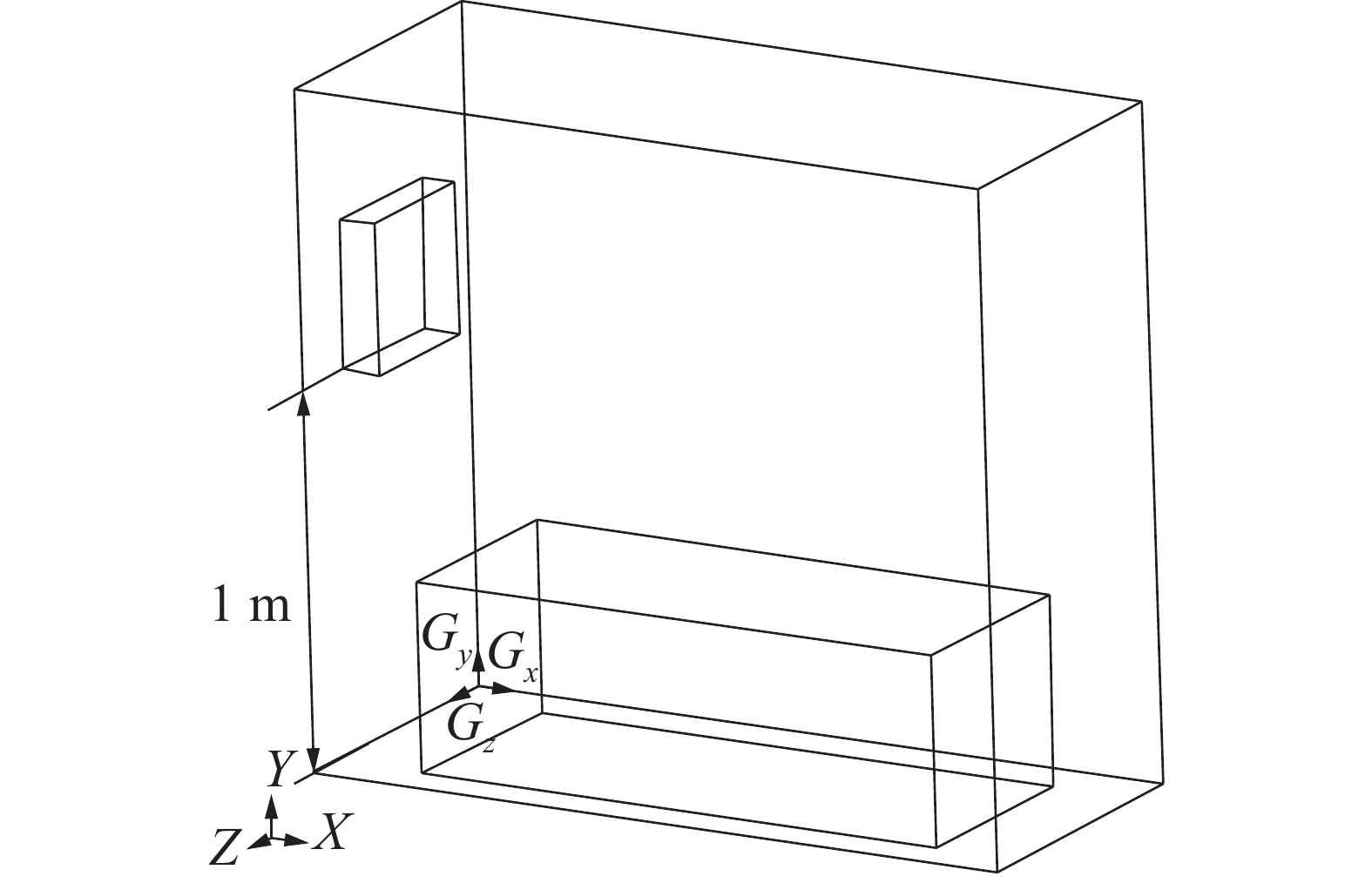Download: 图 8 暖风扇离地1 m时的玻璃温室模型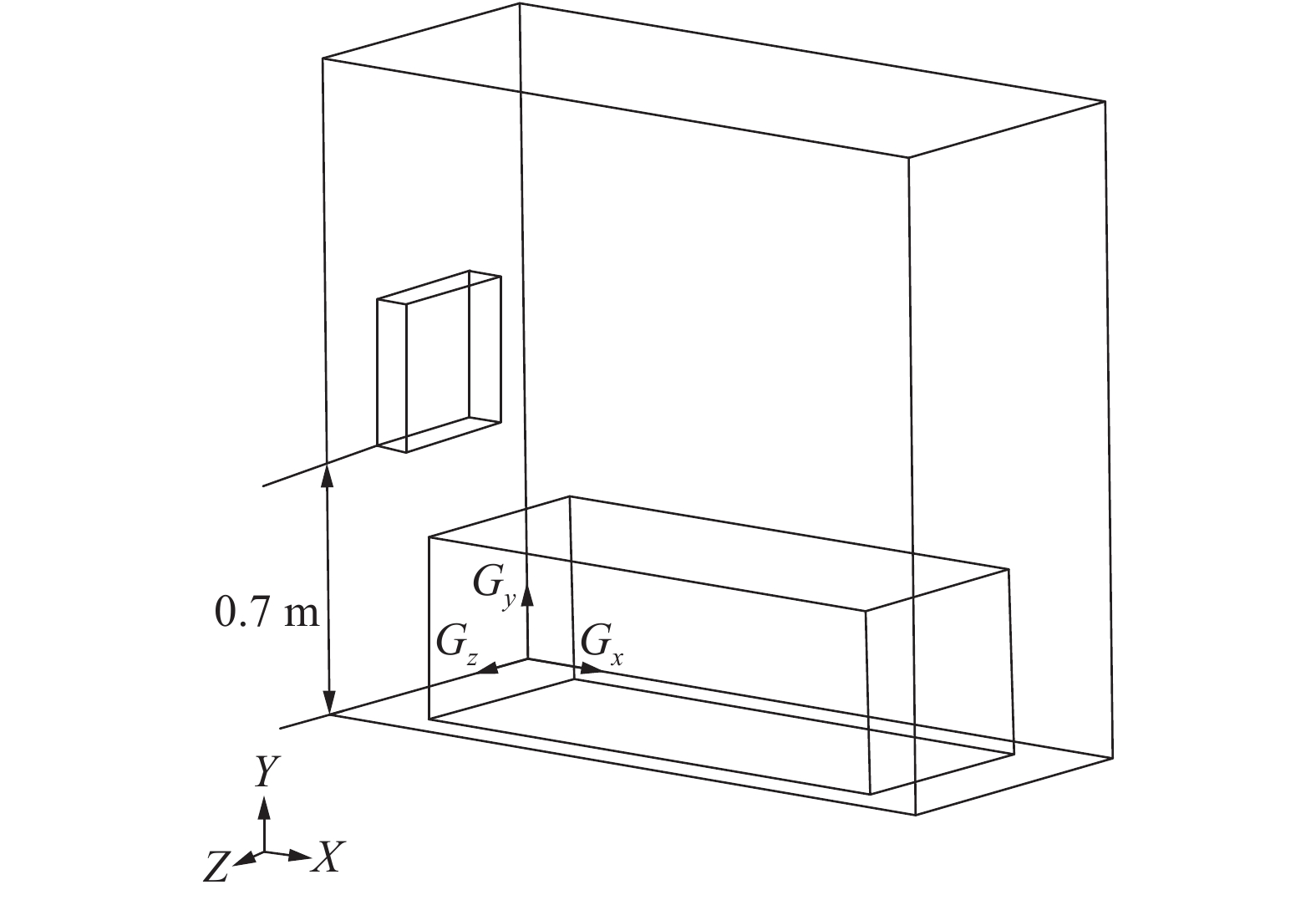Download: 图 9 暖风扇离地0.7 m时的玻璃温室模型
3.2 优化模拟分析 3.2.1 速度场模拟分析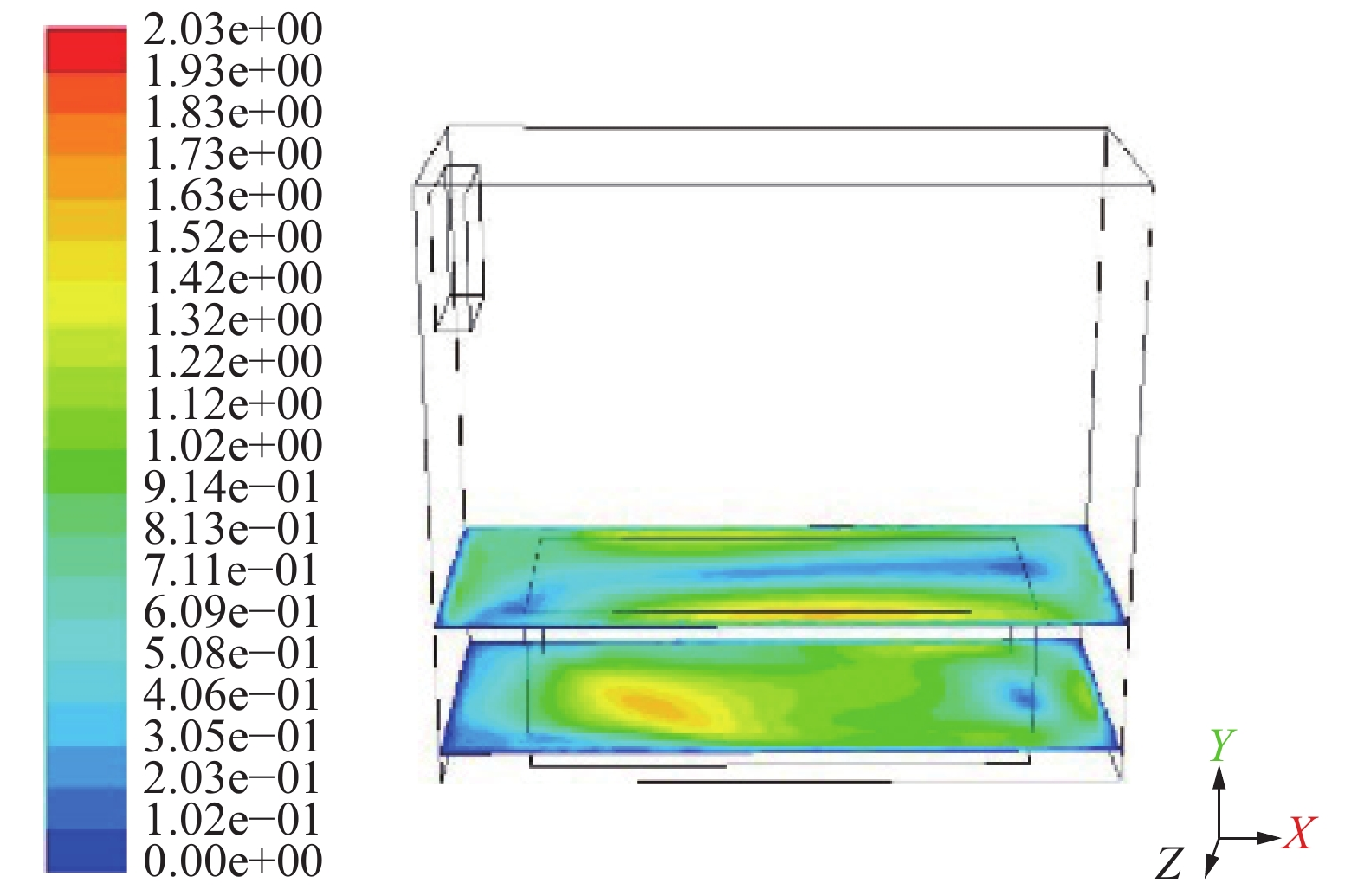Download: 图 10 方案一速度场模拟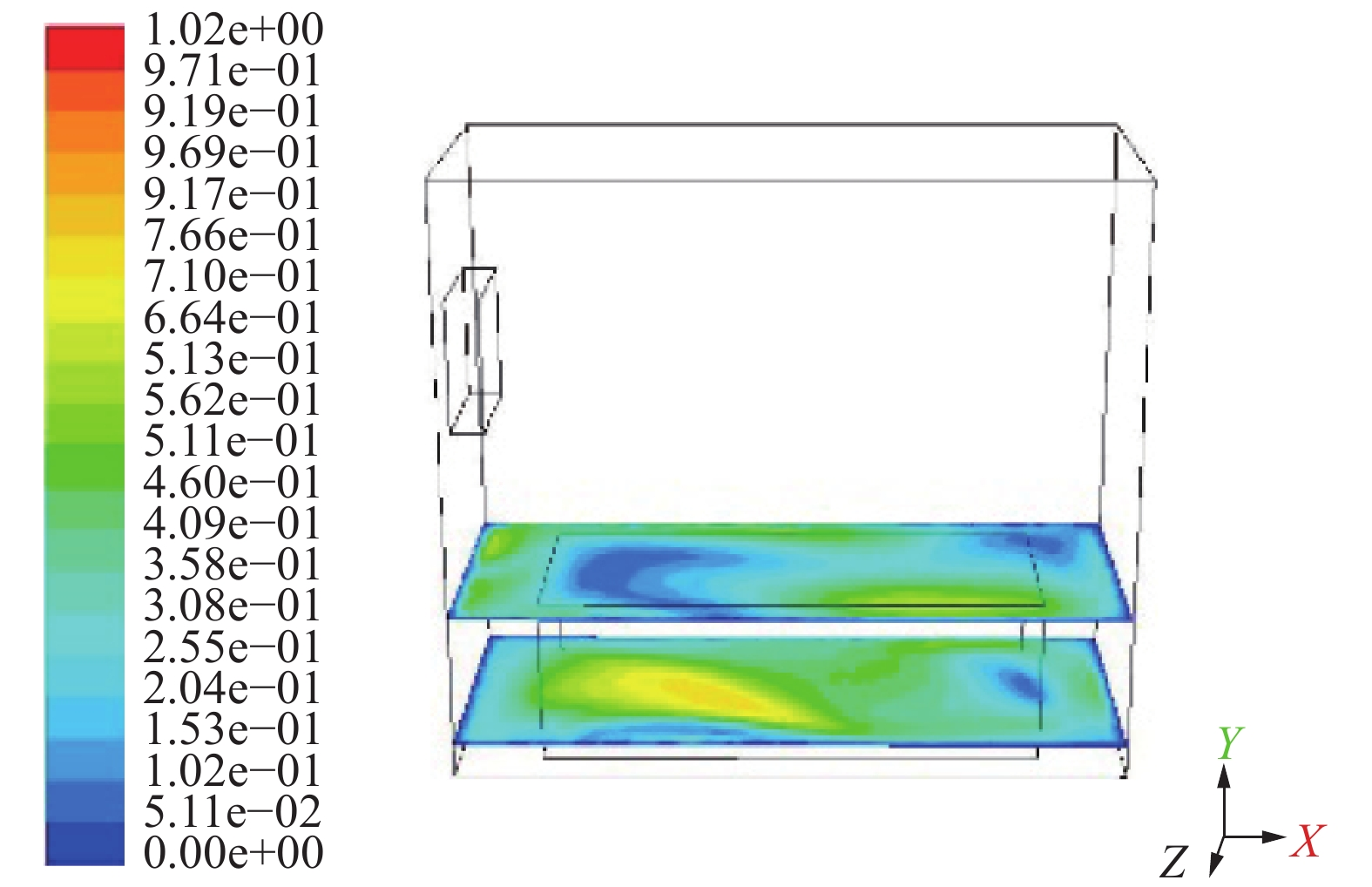Download: 图 11 方案二速度场模拟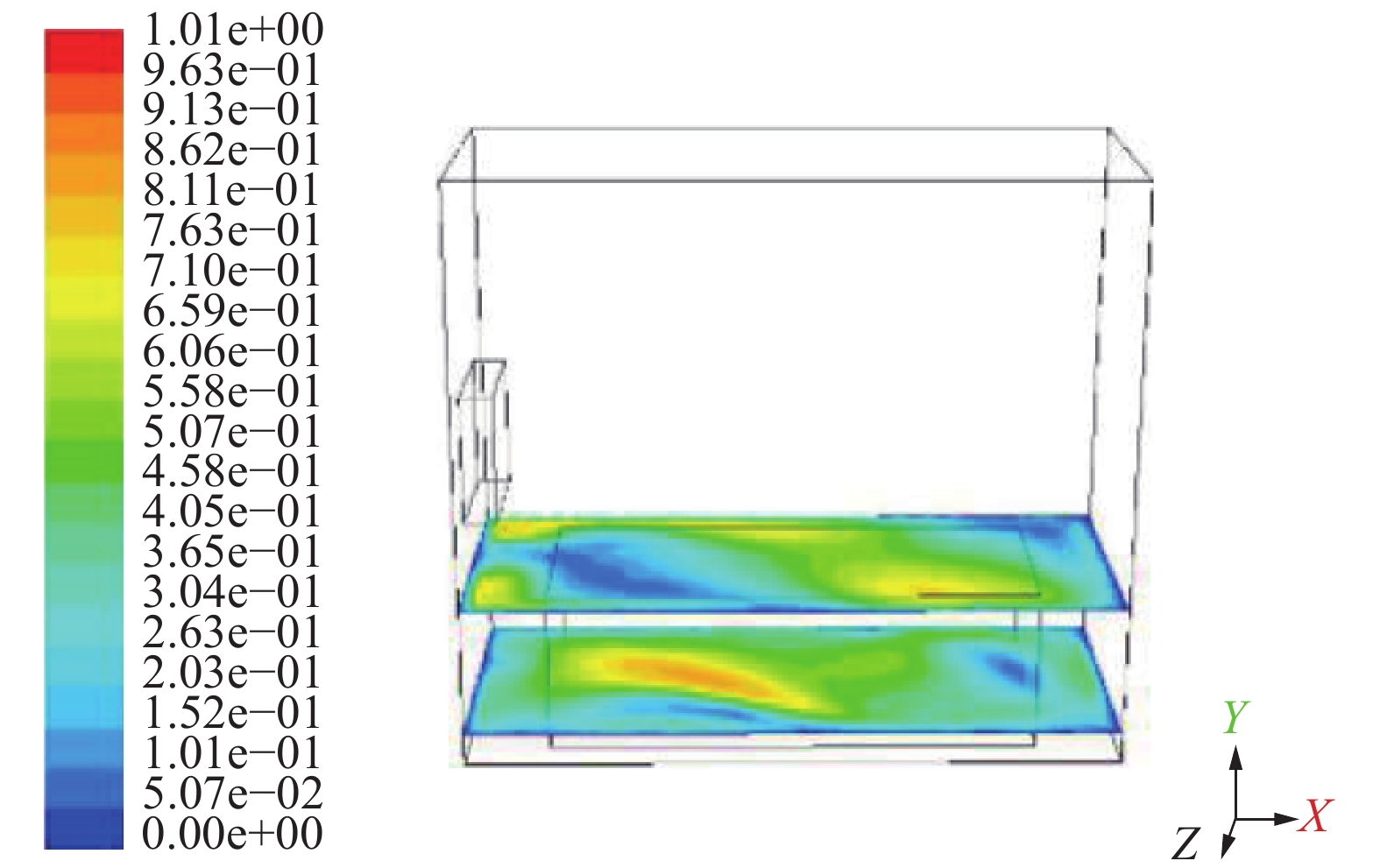Download: 图 12 方案三速度场模拟
3.2.2 温度场模拟分析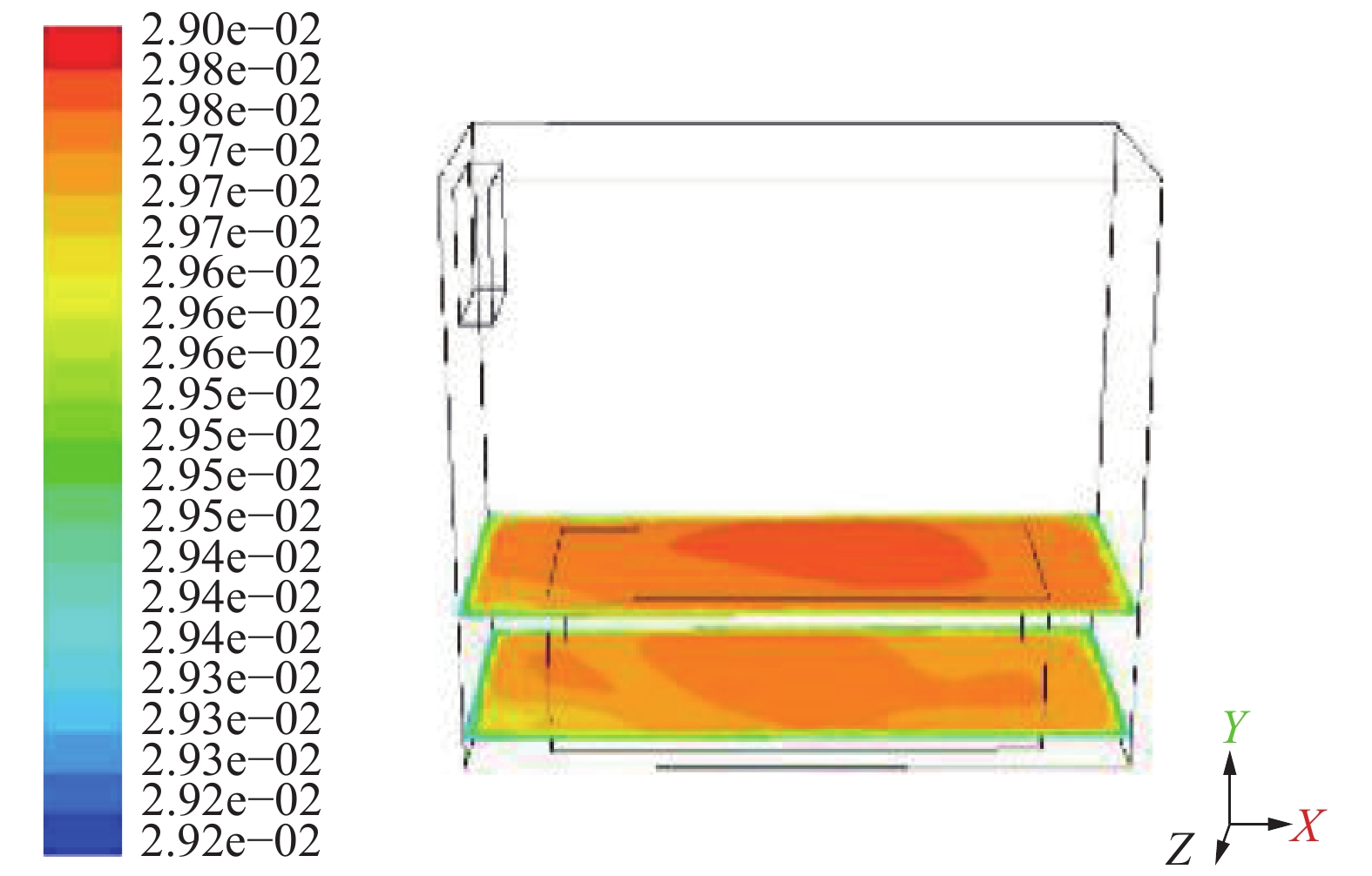Download: 图 13 方案一温度场模拟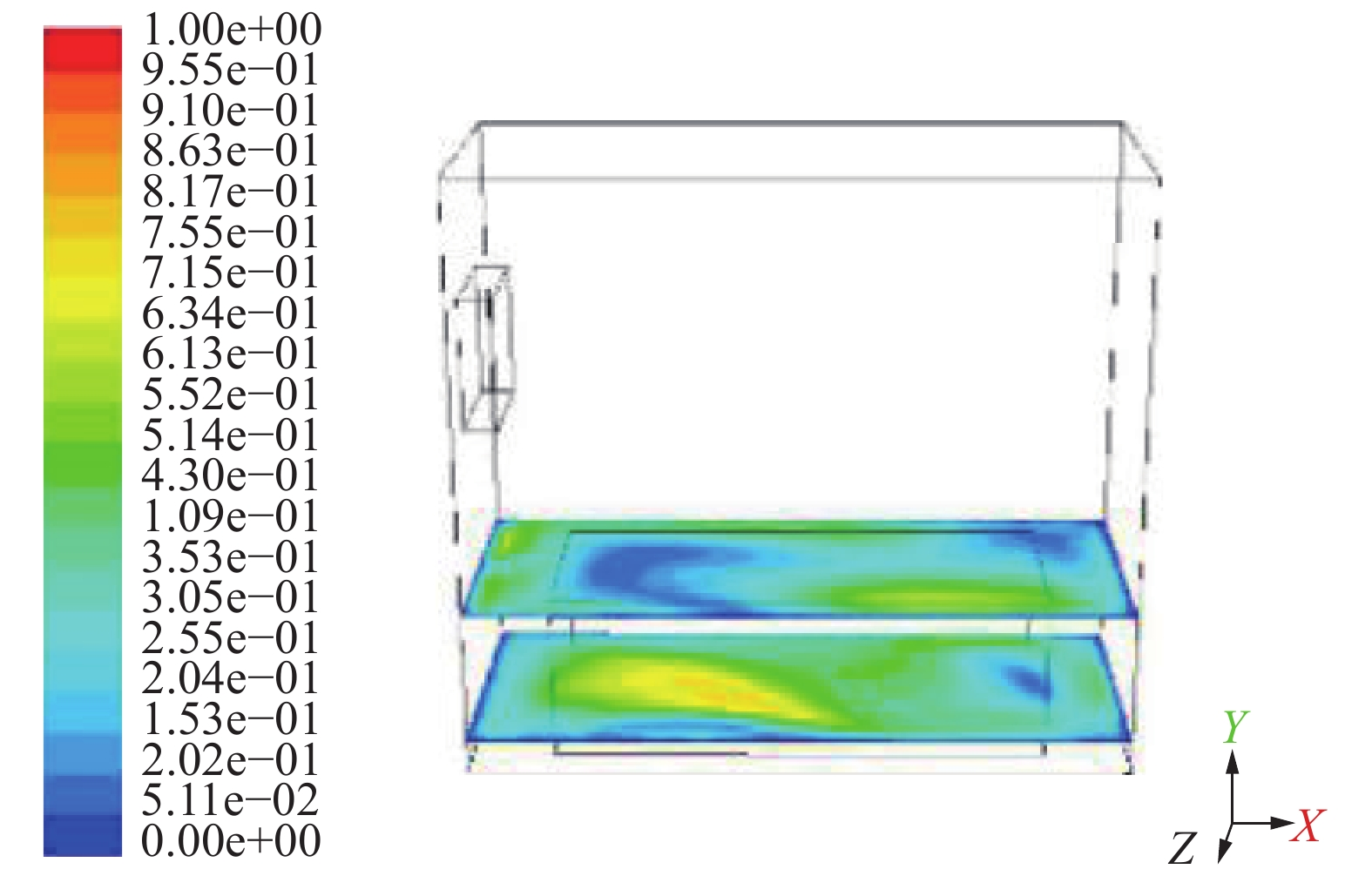Download: 图 14 方案二温度场模拟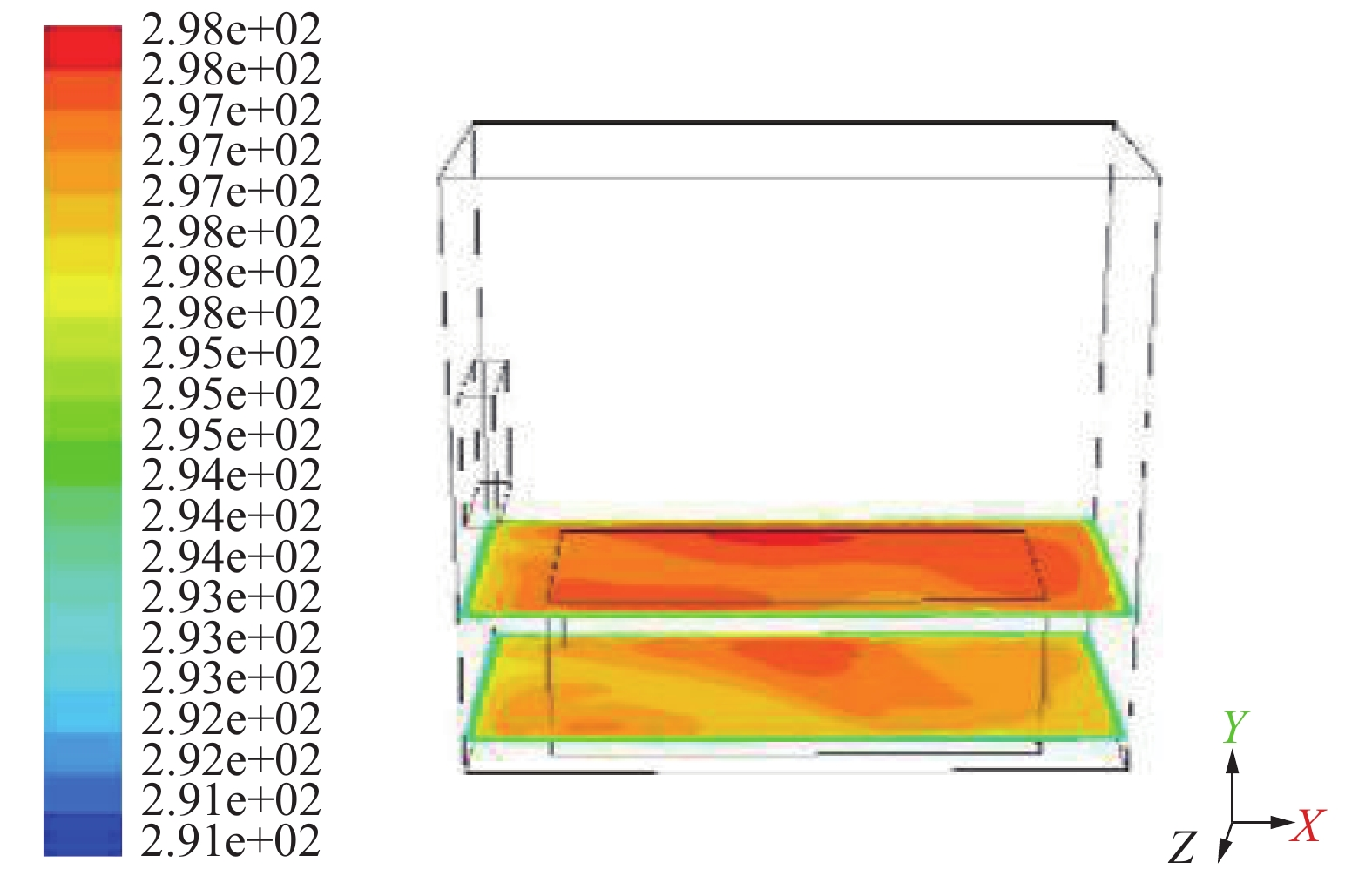Download: 图 15 方案三温度场模拟

3个方案的最高温度分别为24.49、24.45和24.72 ℃，平均温度为23.93、23.15和23.49 ℃，均高于方案零，且植物区域的温度分布均匀性优于方案零。就温度而言，方案一的均值和最大值都为最高，其次为方案三。

4 结论

1）温度数值模拟结果与实验实测结果吻合良好，表明建立的CFD模型有效，模拟玻璃温室内环境分布是可行的；

2）设计的3种结构优化方案的风速值及均匀性均优于方案零。综合考虑，方案三气流循环模式最佳，气流均匀性最好，适宜植物生长。

  程秀花, 毛罕平, 伍德林, 等. 栽有番茄的玻璃温室内气流场分布CFD数值模拟[J]. 江苏大学学报(自然科学版), 2010, 31(5): 510-514. DOI:10.3969/j.issn.1671-7775.2010.05.004 (0)  汪郑邦. 现代温室供热系统的设计及运行模拟研究[D]. 哈尔滨: 哈尔滨工业大学, 2006: 79-80. (0)  刘文合, 杨英英, 徐占洋. 基于CFD方法的温室散热系统结构优化模拟研究[J]. 灌溉排水学报, 2016, 35(4): 8-13. (0)  周伟, 汪小旵, 李永博. 可变边界条件下的Venlo温室温度场三维非稳态模拟[J]. 农业机械学报, 2014, 45(11): 304-310. DOI:10.6041/j.issn.1000-1298.2014.11.047 (0)  张伟建, 王新忠, 李亮亮, 等. 夏季屋顶全开型温室遮阳网降温调控的CFD分析[J]. 江苏农业科学, 2017, 45(19): 253-256. (0)  刘焕. 基于CFD的人工光型植物工厂通风模拟与优化研究[D]. 北京: 中国农业科学院, 2018: 10-29. (0)  段明辉. 冬季温室数值分析研究[D]. 哈尔滨: 东北农业大学, 2014: 7-9. (0)  张艺萌. 基于CFD的寒地水稻育秧大棚环境数值模拟分析与研究[D]. 大庆: 黑龙江八一农垦大学, 2018: 28-54. (0)  宿文, 薛晓萍, 熊宇, 等. 自然通风对日光温室气温影响的模拟分析[J]. 生态学杂志, 2016, 35(6): 1635-1642. (0)  赵杰强, 赵云. 机械通风连栋温室的温度场CFD模拟[J]. 中国农机化学报, 2014, 35(6): 76-79. (0)  何国敏. 现代化温室温度场数字化模拟研究[D]. 南京: 南京农业大学, 2011: 50-67. (0)  沈敏. 食用菌工厂菇房内环境的模拟验证与优化研究[D]. 镇江: 江苏大学, 2016: 23-41. (0)  吴飞青. 温室冬季热环境数值分析与实验研究[D]. 杭州: 浙江工业大学, 2010: 41-71. (0)  刘焕. 基于CFD的人工光型植物工厂通风模拟与优化研究[D]. 北京: 中国农业科学院, 2018: 4. (0)  张芳, 方慧, 杨其长, 等. 基于CFD模型的大跨度温室自然通风热环境模拟[J]. 中国农业气象, 2017, 38(4): 221-229. DOI:10.3969/j.issn.1000-6362.2017.04.003 (0)